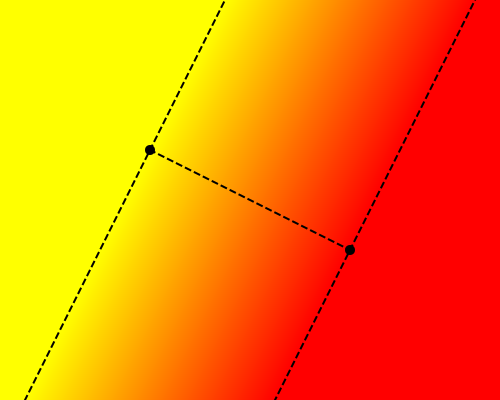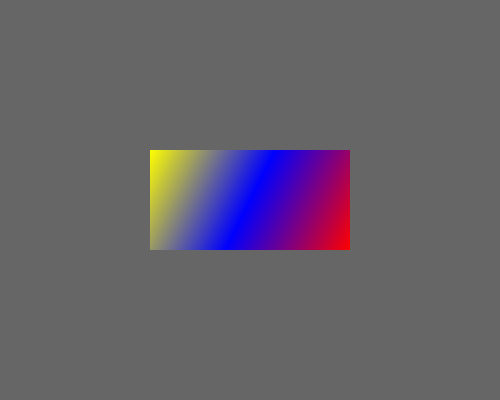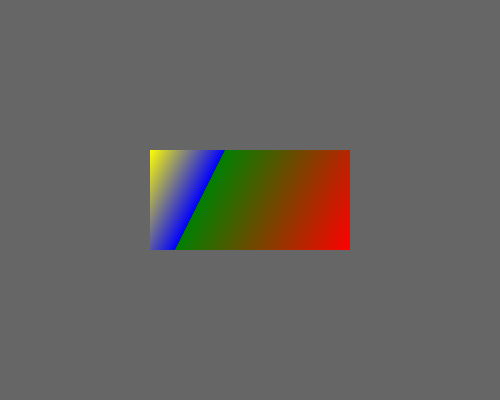Martin McBride, 2021-11-24
Categories generativepy generativepy tutorialThis tutorial covers linear gradients in generativepy.

Linear gradients are a type pattern. Patterns can be used in place of colours to provide alternative fill and stroke styles.

We cover:

• How gradient parameters are defined.

All the code is available on github in tutorial/patterns.

Here is the code for a simple rectangle filled with a gradient:

from generativepy.drawing import make_image, setup
from generativepy.color import Color

def draw(ctx, pixel_width, pixel_height, frame_no, frame_count):

setup(ctx, pixel_width, pixel_height, background=Color(0.4))



This is a fairly simple example of drawing a rectangle. The difference here is that we are filling the rectangle with a gradient rather than a flat colour. Here is the result:The important line is this one:

gradient = LinearGradient().of_points((150, 150), (350, 250)).with_start_end(Color('yellow'), Color('red')).build()


Here is how it works:

• We create a gradient object using LinearGradient.
• We call of_points to specify the endpoints of the gradient. The endpoints are defined in current user space coordinates. We have chosen the corners (top-left and bottom right) of the rectangle we are going to fill.
• with_start_end accepts two colours that represent the start and end colours of the gradient.
• Finally, build builds the object and returns it.

Our gradient starts off yellow (at the top-left of the rectangle), and changes smoothly to red (at the bottom-left of the rectangle). The gradient is diagonal across the rectangle.

To apply the gradient, we simply pass it into the fill method of the rectangle.

## How gradient parameters are defined

The gradient size and shape are defined by two points in the current user space. We chose to use the corners of the rectangle, but the gradient exists over the entire image.

Here is the same gradient, but instead of filling the original rectangle, we will fill a larger rectangle, the size of the full page:On this diagram, the two dots represent the gradient points (150, 150) and (350, 250). The gradient itself fills the band between those two points, which runs perpendicular to the line that joins the two points.

The areas outside this band are filled with a solid colour. The area to the left of the first point is filled with the first colour, yellow. The area to the right of the second point is filled with the second colour, red.

To further illustrate this, here is an image containing several circles, each filled with the gradient above. Notice that each circle is filled with the contents of the gradient for that part of the image (eg the top left circle is yellow, because it is in the area where the gradient is solid yellow, and so on).Here is the code to draw this:

def draw(ctx, pixel_width, pixel_height, frame_no, frame_count):

setup(ctx, pixel_width, pixel_height, background=Color(0.4))



The gradient is only defined once and is used by all the circles.

## Multiple colour changes

The gradient above used two defined colours, yellow at the start point and red at the endpoint. These are often called stops.

It is possible to have more than 2 stops. For example, we could have a gradient that changed from yellow to blue then to red. To do this, we use the with_stops method. This takes a list of stops. Each stop consists of a tuple that indicates the colour and position of the stop. For example:

[(0, Color('yellow')),
(0.5, Color('blue')),
(1, Color('red'))]


This sets the colour to yellow at position 0 (the start of the gradient). It sets the colour to blue at position 0.5 (halfway across the gradient). And it sets the colour to red at position 1 (the end of the gradient). This means that the colour will change from yellow to blue over the first half of the gradient, then from blue to red over the next half. Here is the image created:Here is the code:

def draw(ctx, pixel_width, pixel_height, frame_no, frame_count):

setup(ctx, pixel_width, pixel_height, background=Color(0.4))

.with_stops([(0, Color('yellow')),
(0.5, Color('blue')),
(1, Color('red'))])\
.build()



You can add extra stops, at different positions, to achieve other effects. We will look at a couple of other examples.

This stops list uses the same colour twice:

[(0, Color('yellow')),
(0.3, Color('blue')),
(0.7, Color('blue')),
(1, Color('red'))]


Since the stops at 0.3 and 0.7 are both blue, this means that the colour between these two stops is a flat blue colour. Here is the result:This stops list has two transitions at the same location:

[(0, Color('yellow')),
(0.3, Color('blue')),
(0.3, Color('green')),
(1, Color('red'))]


In this case, the colour goes from yellow to blue to green to red. But the blue and green stops both occur at position 0.3. This means that the gradient varies smoothly from yellow to blue, then at position 0.3 there is a step change to green. The colour then varies smoothly from green to red. Here is the result:If you found this article useful, you might be interested in the book Computer Graphics in Python or other books by the same author.

#### Popular tags

2d arrays abstract data type alignment and angle animation arange arc array arrays behavioural pattern bezier curve built-in function callable object chain circle classes clipping close closure cmyk colour combinations comparison operator comprehension context context manager conversion count creational pattern data science data types design pattern device space dictionary drawing duck typing efficiency ellipse else encryption enumerate fill filter font font style for loop function function composition function plot functools game development generativepy tutorial generator geometry gif global variable gradient greyscale higher order function hsl html image image processing imagesurface immutable object in operator index inner function input installing iter iterable iterator itertools join l system lambda function len line linear gradient linspace list list comprehension logical operator lru_cache magic method mandelbrot mandelbrot set map matplotlib monad mutability named parameter numeric python numpy object open operator optimisation optional parameter or pandas partial application path pattern permutations polygon positional parameter print pure function python standard library radial gradient range recipes rectangle recursion reduce repeat rgb rotation roundrect scaling scipy sector segment sequence setup shape singleton slice slicing sound spirograph sprite square str stream string stroke structural pattern subpath symmetric encryption template text text metrics tinkerbell fractal transform translation transparency triangle truthy value tuple turtle unpacking user space vectorisation webserver website while loop zip